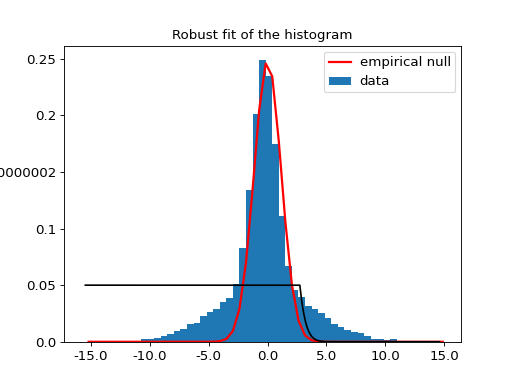Empirical null¶

The nipy.algorithms.statistics.empirical_pvalue module contains a class that fits a Gaussian model to the central part of an histogram, following Schwartzman et al, 2009. This is typically necessary to estimate a FDR when one is not certain that the data behaves as a standard normal under H_0.

The NormalEmpiricalNull class learns its null distribution on the data provided at initialisation. Two different methods can be used to set a threshold from the null distribution: the NormalEmpiricalNull.threshold() method returns the threshold for a given false discovery rate, and thus accounts for multiple comparisons with the given dataset; the NormalEmpiricalNull.uncorrected_threshold() returns the threshold for a given uncorrected p-value, and as such does not account for multiple comparisons.

Example¶

If we use the empirical normal null estimator on a two Gaussian mixture distribution, with a central Gaussian, and a wide one, it uses the central distribution as a null hypothesis, and returns the threshold following which the data can be claimed to belong to the wide Gaussian:

# emacs: -*- mode: python; py-indent-offset: 4; indent-tabs-mode: nil -*-
# vi: set ft=python sts=4 ts=4 sw=4 et:
import numpy as np

from nipy.algorithms.statistics.empirical_pvalue import NormalEmpiricalNull

x = np.c_[np.random.normal(size=10000),
np.random.normal(scale=4, size=10000)]

enn = NormalEmpiricalNull(x)
enn.threshold(verbose=True)The threshold evaluated with the NormalEmpiricalNull.threshold() method is around 2.8 (using the default p-value of 0.05). The NormalEmpiricalNull.uncorrected_threshold() returns, for the same p-value, a threshold of 1.9. It is necessary to use a higher p-value with uncorrected comparisons.

Class documentation¶

class nipy.algorithms.statistics.empirical_pvalue.NormalEmpiricalNull(x)

Class to compute the empirical null normal fit to the data.

The data which is used to estimate the FDR, assuming a Gaussian null from Schwartzmann et al., NeuroImage 44 (2009) 71–82

__init__(x)

Initialize an empirical null normal object.

Parameters
x1D ndarray

The data used to estimate the empirical null.

fdr(theta)

Given a threshold theta, find the estimated FDR

Parameters
thetafloat or array of shape (n_samples)

values to test

Returns
afpvalue of array of shape(n)
fdrcurve()

Returns the FDR associated with any point of self.x

learn(left=0.2, right=0.8)

Estimate the proportion, mean and variance of a Gaussian distribution for a fraction of the data

Parameters
left: float, optional

Left cut parameter to prevent fitting non-gaussian data

right: float, optional

Right cut parameter to prevent fitting non-gaussian data

Notes

This method stores the following attributes:

• mu = mu

• p0 = min(1, np.exp(lp0))

• sqsigma: variance of the estimated normal distribution

• sigma: np.sqrt(sqsigma) : standard deviation of the estimated normal distribution

plot(efp=None, alpha=0.05, bar=1, mpaxes=None)

Plot the histogram of x

Parameters
efpfloat, optional

The empirical FDR (corresponding to x) if efp==None, the false positive rate threshold plot is not drawn.

alphafloat, optional

The chosen FDR threshold

bar=1bool, optional
mpaxes=None: if not None, handle to an axes where the fig
will be drawn. Avoids creating unnecessarily new figures
threshold(alpha=0.05, verbose=0)

Compute the threshold corresponding to an alpha-level FDR for x

Parameters
alphafloat, optional

the chosen false discovery rate threshold.

verboseboolean, optional

the verbosity level, if True a plot is generated.

Returns
theta: float

the critical value associated with the provided FDR

uncorrected_threshold(alpha=0.001, verbose=0)

Compute the threshold corresponding to a specificity alpha for x

Parameters
alphafloat, optional

the chosen false discovery rate (FDR) threshold.

verboseboolean, optional

the verbosity level, if True a plot is generated.

Returns
theta: float

the critical value associated with the provided p-value

Reference: Schwartzmann et al., NeuroImage 44 (2009) 71–82